# Quiz 8: Game Theory

Business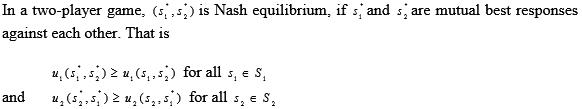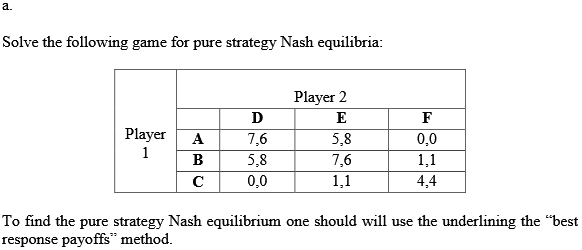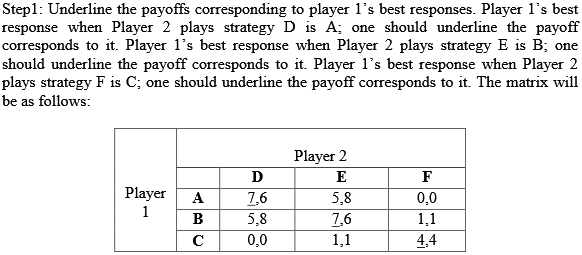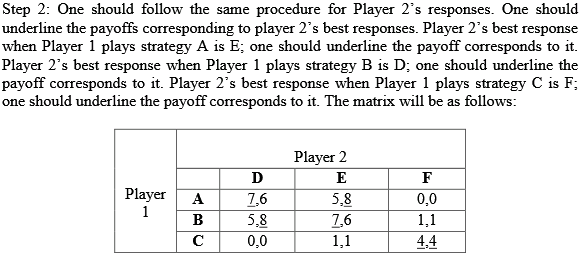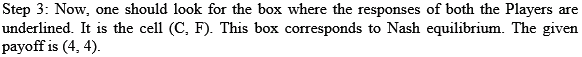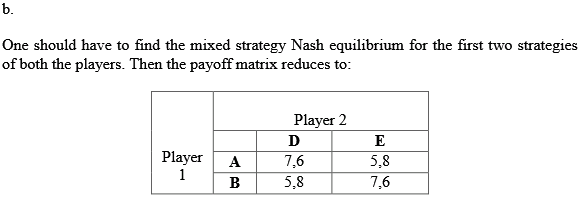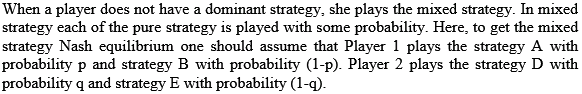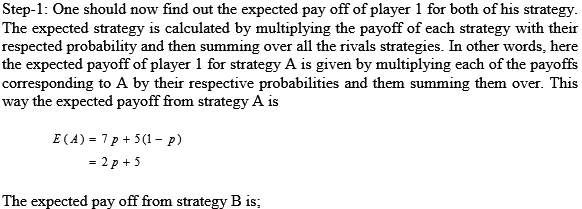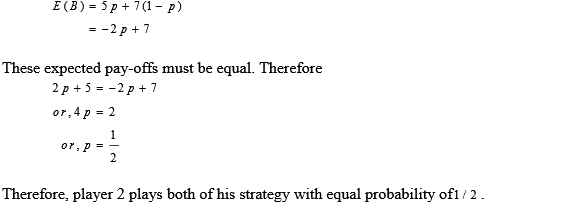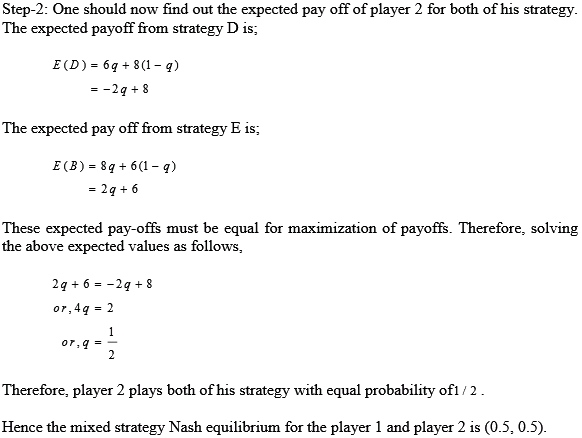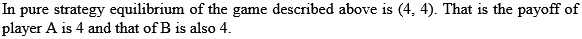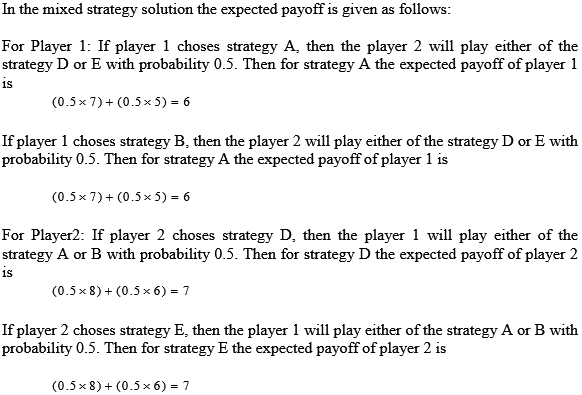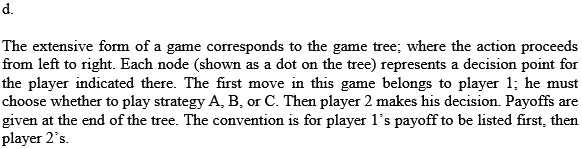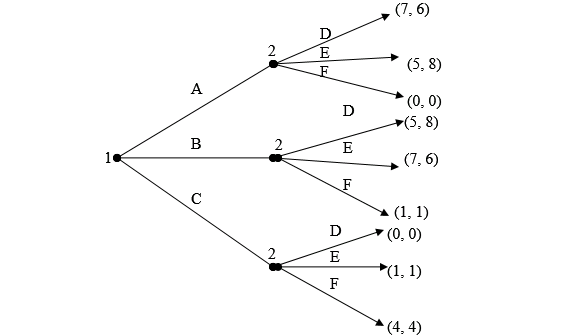There is no answer for this question

The Nash equilibrium is a strategy profile in which each player plays his best response given other player's strategy and no unilateral transfer can have made any player better off. The payoffs of the chicken game is given. The two teens are the players and their strategies are to veer off or does not veer off. Their respective payoffs are specified in the table. a. The extensive form of the game is used to model one shot game in a tree diagram where each player is choosing strategies simultaneously.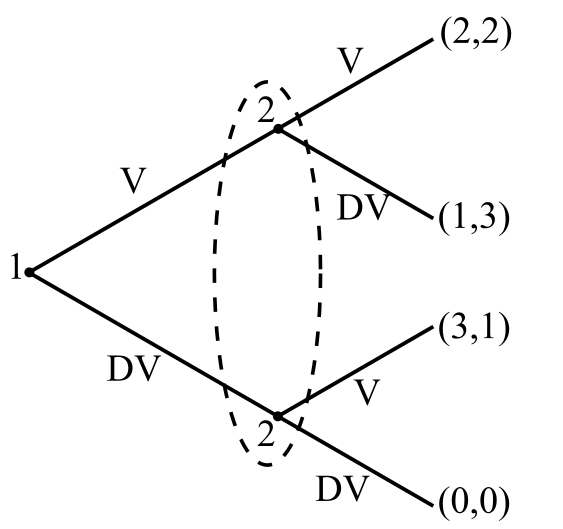b. Pure strategy Nash equilibria refers to those payoffs which are best response of each player with respect to other player's strategy and no player has a reason to deviate from the Nash equilibrium strategy. The best response for each player given other player's strategy is indicated by a line in the table given below and the outcome which has both payoffs underlined will give the pure strategy Nash equilibrium.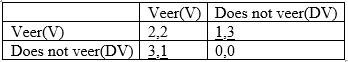From the table above, the pure strategy Nash equilibria are (does not veer, veer) and (does not veer, does not veer). c. Mixed strategy Nash equilibrium is a probability distribution which is chosen by each player which is best for him given the strategies used by the other players. The expected payoffs of player one given mixed strategy of player two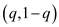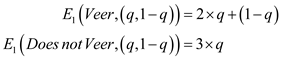For player one to be indifferent between the two strategies in equilibrium requiresSimilarly, expected payoffs of player two can be derived given mixed strategy of player one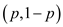and equating to derive equilibrium value of probability as done below:To depict the best response functions diagrammatically, it is required to determine the relation between p and q based on their respective payoffs.Similarly,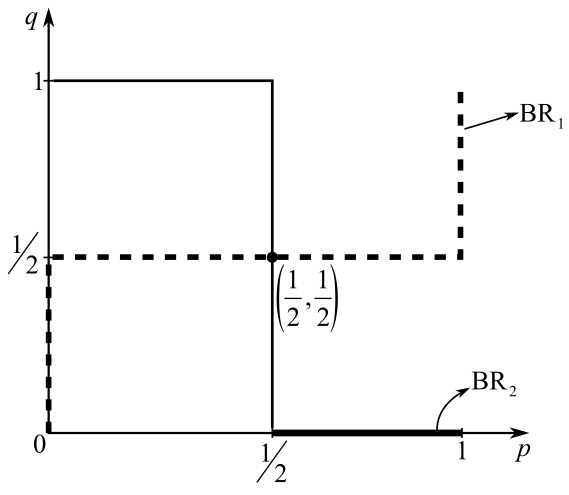From the above figure, it is clear that the mixed strategy Nash equilibrium is the point where best response curves of both players intersect. Both players have symmetric Nash equilibrium as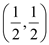. d. Suppose the game is played sequentially with player one making the first move and choosing his actions, the contingent strategy of player two will be seen as done below: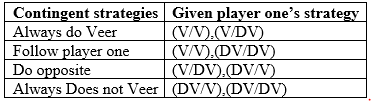The normal form for the sequential game can be seen below: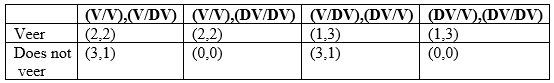The extensive form of the game can be seen diagrammatically as done below: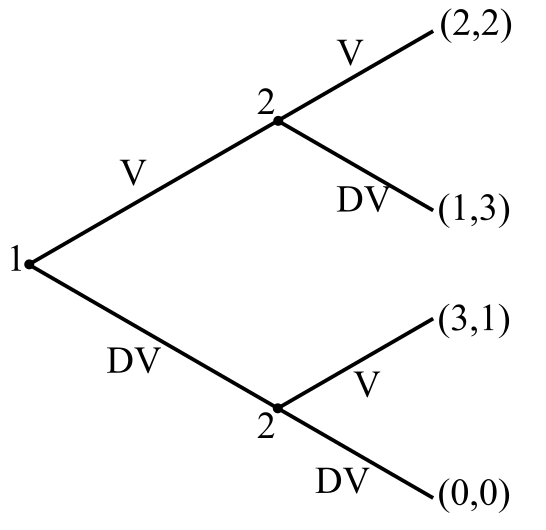e. It is required to solve for Nash equilibria using the normal form. The normal form of the game is seen as done below: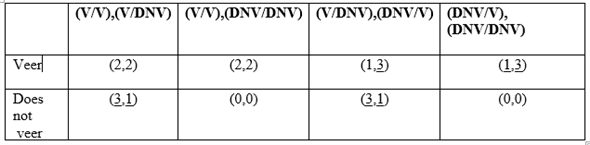It is important to note that Does not veer can be written as DNV or DV. The best response for each player given other player's strategy is indicated by a line in the table given below and the outcome which has both payoffs underlined will give the pure strategy Nash equilibrium. Hence, the sequential game reveals three pure strategy Nash equilibria as 1. Player one plays DOES NOT VEER, player two plays (V/V), (V/DNV). 2. Player one plays VEER, player two plays (DNV/V), (DNV/DNV) 3. Player one plays DOES NOT VEER, Player two plays (DNV/V), (DNV/V) f. Sub game perfect equilibrium is a Nash equilibrium for each subgame in a sequential game. Every finite sequential game pure strategy Nash equilibrium can be derived using backward induction. Backward induction is a process of reasoning backwards in time. From the end, to determine a sequence of optimal actions. The sub games in the given chicken game can be seen as done below: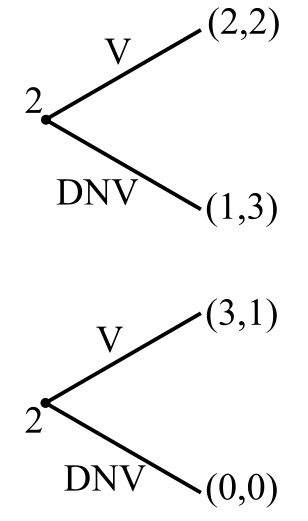Using backward induction, first determine the best response of player two in both the subgames as player two is playing in the last stage of the game. After getting the optimal actions, move to player one and determine his optimal action based on the given optimal strategy of his opponent.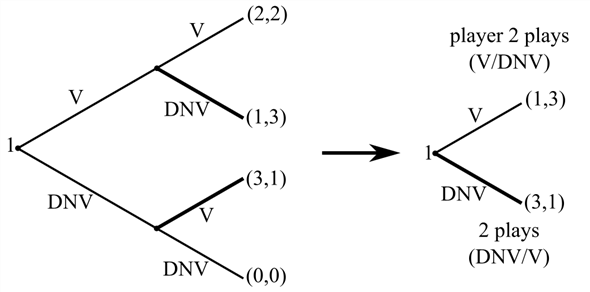From backward induction, the subgame perfect equilibrium is Player one plays DOES NOT VEER, Player two plays (DNV/V), (DNV/V). It rules out the empty threats and only shows the equilibrium path. Threats to play irrationally or choose something other than the best response is ruled out. There are credible threats which should considered and empty threats should be neglected when considering the equilibrium path. The other two strategies which were part of normal extensive game pure strategy Nash equilibrium fall prey to empty threats and lie off the equilibrium path.